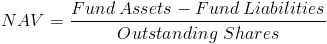# Net Asset ValueThe net asset value formula is used to calculate a mutual fund's value per share. A mutual fund is a pool of investments that are divided into shares to be purchased by investors. Each share contains a weighted portion of each investment in the collective pool. The premise of grouping in this manner is to minimize risk by diversifying.

It is important to note that net asset value does not look at future dividends and growth as do other stock and bond valuation methods. The formula for net asset value only looks at the fund's per share value based on its net assets.

The net asset value is determined by the mutual fund company and priced according to this formula. Stock and bond valuation methods are not used due to mutual funds being sold directly from the company and not through an exchange or on the secondary market. Stocks, on the other hand, are sold through bid and ask pricing on the secondary market which requires an investor to determine a share's value to them based on expected future earnings, in which they bid accordingly.

## Use of the Net Asset Value Formula

As already stated, the net asset value formula is used by a mutual fund company to determine the price of a share of a specific mutual fund. An individual investor may not find the net asset value formula particularly useful besides for the sake of knowledge. However, it is important to know how net asset value is calculated, in that expected future earnings are not considered.

## Example of Net Asset Value Formula

A simple, perhaps unrealistic, example of calculating net asset value would be a mutual fund with assets of \$1 million, liabilities of \$100,000, and 100,000 outstanding shares. Putting this information into the variables of the net asset value formula would showwhich would return \$9 per share.

New to Finance?